Courses

# Test: Fluid Mechanics- 1

## 30 Questions MCQ Test Physics For JEE | Test: Fluid Mechanics- 1

Description
This mock test of Test: Fluid Mechanics- 1 for Class 11 helps you for every Class 11 entrance exam. This contains 30 Multiple Choice Questions for Class 11 Test: Fluid Mechanics- 1 (mcq) to study with solutions a complete question bank. The solved questions answers in this Test: Fluid Mechanics- 1 quiz give you a good mix of easy questions and tough questions. Class 11 students definitely take this Test: Fluid Mechanics- 1 exercise for a better result in the exam. You can find other Test: Fluid Mechanics- 1 extra questions, long questions & short questions for Class 11 on EduRev as well by searching above.
QUESTION: 1

### A bucket contains water filled upto a height = 15 cm. The bucket is tied to a rope which is passed over a frictionless light pulley and the other end of the rope is tied to a weight of mass which is half of that of the (bucket + water). The water pressure above atmosphere pressure at the bottom is :

Solution:

Consider the mass of the block be m, thus we get a mass of bucket + water is 2m. Now if we consider it as a two mass pulley, we get  the net acceleration a by which the bucket is going down can be found by -
2mg - mg = 3ma
Thus we get a = g/3
Now we know that the bucket is accelerating with acceleration g/3 downwards hence to treat it as an inertial system we need to apply pseudo force upon it in upward direction. Thus we get in reference to the bucket net acceleration is 2g/3 downwards. Thus the pressure at the bottom is P + x 0.15 x 2g/3 = P + 1 kPa

QUESTION: 2

Solution:
QUESTION: 3

### A liquid of mass 1 kg is filled in a flask as shown in figure. The force exerted by the flask on the liquid is (g = 10 m/s2) [Neglect atmospheric pressure]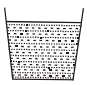Solution:

The force exerted by the flas on the liquid is equal to the force applied by the liquid on the flask which is equal to its weight = mg = 10N

QUESTION: 4

A U-tube having horizontal arm of length 20 cm, has uniform cross-sectional area = 1 cm2. It is filled with water of volume 60 cc. What volume of a liquid of density 4 g/cc should be poured from one side into the U-tube so that no water is left in the horizontal arm of the tube?

Solution: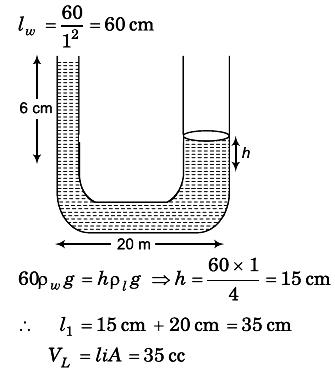QUESTION: 5

A light semi cylindrical gate of radius R is piovted at its mid point O, of the diameter as shown in the figure holding liquid of density r. The force F required to prevent the rotation of the gate is equal to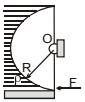Solution:

The force is normal to the surface of the cylinder and hence will pass through the centre. And since the forces pass through the centre it means that the net torque will be zero.

QUESTION: 6

The pressure at the bottom of a tank of water is 3P where P is the atmospheric pressure. If the water is drawn out till the level of water is lowered by one fifth., the pressure at the bottom of the tank will now be

Solution:
QUESTION: 7

An open-ended U-tube of uniform cross-sectional area contains water (density 1.0 gram/centimeter3) standing initially 20 centimeters from the bottom in each arm. An immiscible liquid of density 4.0 grams/centimeter3 is added to one arm until a layer 5 centimeters high forms, as shown in the figure above. What is the ratio h2/h1 of the heights of the liquid in the two arms ?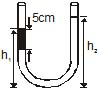Solution:

5 × 4 × 10 + (h1 - 5) × 10 = h2 × 1 × 10
200 + 10h1 – 50 = 10h2
10h2 - 10h1 = 150
h2 - h1 = 15
h2 + h1 = 20 + 20 + 5 = 45
2h2 = 60
h2 = 30
h1 = 15
h2/h1 = 30/15
h2/h1 = 2/1

QUESTION: 8

The area of cross-section of the wider tube shown in figure is 800 cm2. If a mass of 12 kg is placed on the massless piston, the difference in heights h in the level of water in the two tubes is :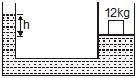Solution:

h × ρ × 10 = 120/800 × 10-4
h × ρ = 12/8 × 10
h × ρ = 150m
h × 1000 = 150m
h = 15cm

QUESTION: 9

A body is just floating in a liquid (their densities are equal) If the body is slightly pressed down and released it will -

Solution:

The body will sink to the bottom as it gains a downward velocity and has no force to bring it back up. The weight becomes greater than upwards thrust.

QUESTION: 10

Two stretched membranes of areas 2 and 3 m2 are placed in a liquid at the same depth. The ratio of the pressure on them is -

Solution:

Pressure depends on depth of container irrespective of its shape and in the above case depth for both the vessels are given same. Therefore the hydraulic pressure for the containers will be in the ratio 1: 1

QUESTION: 11

An ice block floats in a liquid whose density is less than water. A part of block is outside the liquid. When whole of ice has melted, the liquid level will -

Solution:

Ice is lighter than water. When ice melts, the volume occupied by water is less than that of ice, due to which the level of water goes down.

QUESTION: 12

A fluid container is containing a liquid of density r is is accelerating upward with acceleration a along the inclined place of inclination a as shwon. Then the angle of inclination q of free surface is :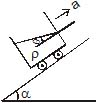Solution:

First resolve all components in the along and perpendicular to incline. Pressure difference is created in a vertical column full of liquid. This is because of gravity acting in downward direction. Similarly, pressure difference will be created too along the incline. So, p = h * d * g * cosa (in perpendicular direction) and
p = hd (a + g sina) (along incline).
So, tan(theta) = (a + gsina)/(gcosa)

QUESTION: 13

Figure shows a three arm tube in which a liquid is filled upto levels of height l. It is now rotated at an angular frequency w about an axis passing through arm B. The angular frequency w at which level of liquid of arm B becomes zero.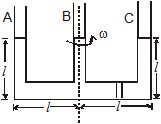Solution: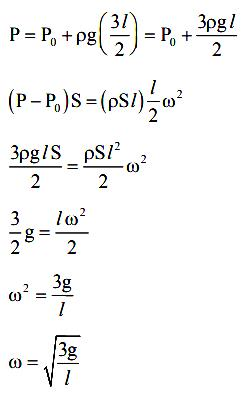QUESTION: 14

Two cubes of size 1.0 m sides, one of relative density 0.60 and another of relative density = 1.15 are connected by weightless wire and placed in a large tank of water. Under equilibrium the lighter cube will project above the water surface to a height of

Solution: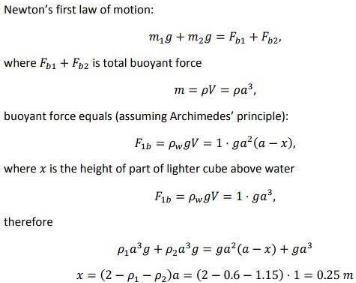which is equal to 25 cm.

QUESTION: 15

A cuboidal piece of wood has dimensions a, b and c. Its relative density is d. It is floating in a larger body of water such that side a is vertical. It is pushed down a bit and released. The time period of SHM executed by it is :

Solution:

Time period of SHM of small vertical oscillations in a liquid is given by T=2π√l/g, where l is the length of cube/cylinder dipped in the water.
So according to law of floatation,
weight of the cube = weight of the water displaced
abc × d × g=bcl × 1×g
⇒l=da
⇒T=2π√da/g

QUESTION: 16

Two bodies having volumes V and 2V are suspended from the two arms of a common balance and they are found to balance each other. If larger body is immersed in oil (density d1 = 0.9 gm/cm3) and the smaller body is immersed in an unknown liquid, then the balance remain in equilibrium. The density of unknown liquid is given by :

Solution:
QUESTION: 17

A boy carries a fish in one hand and a bucket (not full) of water in the other hand. If the places the fish in the bucket, the weight now carried by him (assume that water does not spill) :

Solution:

Buoyant force on fish due to water will be equal and opposite to the force on water by fish.
These two forces are internal forces for the fish bucket system.
Hence, they will not affect the weight the boy carries.

QUESTION: 18

A piece of steel has a weight W in air, W1 when completely immersed in water and W2 when completely immersed in an unknown liquid. The relative density (specific gravity) of liquid is :

Solution:

If the loss of weight of a body in water is 'a' while in liquid is 'b' then
Sigma in liquid / sigma in water = upthrust on body in liquid / upthrust on body in water
Then a / b = (W air - W liquid) / (W air - W water).

QUESTION: 19

A ball of relative density 0.8 falls into water from a height of 2m. The depth to which the ball will sink is (neglect viscous forces) :

Solution: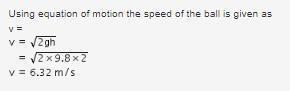Let us calculate the buoyancy force by water try to stop the ball.
Buoyancy force = weight of displaced water
= Density of water x Volume of the ball x g
= d x V x g  --- (1)
But buoyant force = ma
Therefore, ma = d x V x g
or a = (dVg) / m --- (2)
Let the density of the ball be d'.
→ m = d'V
Substituting in equation 2, we get
a = (dVg) / d'V
= dg / d'
= (d/d') x g
Given that relative density, (d / d') = 0.8
So, a = g / (0.8)
= 10 / 0.8
→ a = 12.5 m/s^2
Net deceleration of ball, a' = a - g = 12.5 - 10
= 2.5 m/s^2
Final speed of ball v' = 0
Using the equation - v'^2 = v^2 + 2a's (where s = depth of ball in the water)
Substituting the values in the above equation, we get
40 = 0 + 2 x 2.5 x s
s = 8m

QUESTION: 20

A cube of iron whose sides are of length L, is put into mercury. The weight of iron cube is W. The density of iron is rI, that of mercury is rM. The depth to which the cube sinks is given by the expression _

Solution:
QUESTION: 21

A metal ball of density 7800 kg/m3 is suspected to have a large number of cavities. It weighs 9.8 kg when weighed directly on a balance and 1.5 kg less when immersed in water. The fraction by volume of the cavities in the metal ball is approximately :

Solution:

Here when put in water the water displaces/fills the empty cavity.
Volume of only metal = mass/density = 9.8/7800 = 1.25X10-3 cubic m
Volume of whole ball including cavity = weight of water displaced/density of water
Density of water is 1000 kg/cubic m.
Volume of whole ball = 1.5X10-3 cubic m
Volume of cavity = Volume of ball - volume of metal
= 1.5X10-3 - 1.25X10-3
= 0.25X10-3 cubic m
Ratio of volume of cavity/ball = 0.25X10^-3 / 1.5X10^-3 = 0.16 = 16%
0.16 fraction of whole ball is a cavity i.e. 16%

QUESTION: 22

A sphere of radius R and made of material of relative density s has a concentric cavity of radius r. It just floats when placed in a tank full of water. The value of the ratio R/r will be

Solution:

If the sphere is "just" floating means the sphere is exactly immersed in water (or) the buoyant force acting on it is exactly equal to its weight. The cavity reduces the weight of the actual sphere but has no role in the buoyant force.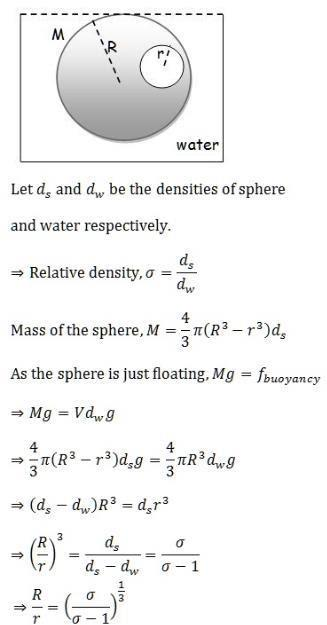QUESTION: 23

A beaker containing water is placed on the platform of a spring balance. The balance reads 1.5 kg. A stone of mass 0.5 kg and density 500 kg/m3 is immersed in water without touching the walls of beaker. What will be the balance reading now ?

Solution:

Since the weight of the block must be equal to the buoyant force acting on the block for it to remain in equilibrium,

B=0.5kg

The reading of the spring balance = Weight of water + Buoyant force' reaction pair force downwards

=1.5kg+0.5kg=2kg

QUESTION: 24

A cylindrical block of area of cross-section A and of material of density r is placed in a liquid of density one-third of density of block. The block compresses a spring and compression in the spring is one-third of the length of the block. If acceleration due to gravity is g, the spring constant of the spring is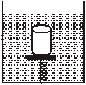Solution:

Since the block is at rest, net force on the cylinderical block is zero, ie net upward = net downward force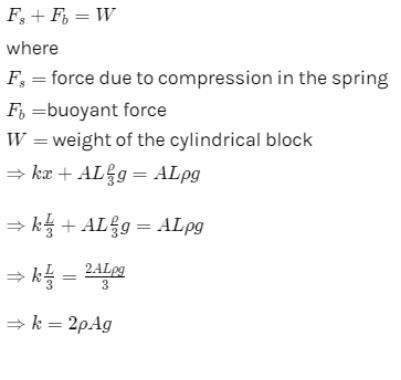QUESTION: 25

A body of density r' is dropped from rest at a height h into a lake of density r, where r > r'. Neglecting all disipative froces, calculate the maximum depth to which the body sinks before returning of float on the surface.

Solution:
QUESTION: 26

Water is flowing in a horizontal pipe of

non-uniform cross - section. At the most contracted place of the pipe –

Solution:

Continuity equation states that, "For a non-viscous liquid and streamlined flow the volume flow rate (Area of cross section x velocity) is constant throughout the flow at any point".
According to this, Av = constant. So if at any point the cross-section area decreases then velocity of liquid at that point increases and vice-versa.
Bernoulli's equation states that, "For a streamlined and non-viscous flow the total energy (kinetic energy and pressure gradient) remains constant throughout the liquid.
According to this, kinetic energy + Pressure gradient = constant. So, if at any point the velocity increases the pressure at that point decreases and vice-versa.
At the most contracted place of the pipe area of cross section is minimum
⇒ velocity is maximum
⇒ pressure is minimum

QUESTION: 27

Water is flowing in a tube of non-uniform radius. The ratio of the radii at entrance and exit ends of tube is 3 : 2. The ratio of the velocities of water entering in and exiting from the tube will be –

Solution:

We know, for the fluid flowing through the non-uniform pipe the velocity of fluid is inversely proportional to the area of cross-section.
Hence, if v1, v2 are the velocities of entry and exit end of the pipe and a1, a2 are the area of cross-sections of entry and exit end of the pipe, then
v1/v2=a2/a1
⇒v1/v2​=(r2)2/(r1)2
∴v1/v2​=(2)2/(3)2​=4/9​

QUESTION: 28

A rectangular tank is placed on a horizontal ground and is filled with water to a height H above the base. A small hole is made on one vertical side at a depth D below the level of the water in the tank. The distance x from the bottom of the tank at which the water jet from the tank will hit the ground is

Solution:

Time taken by liquid drop to fall
S=ut+(1/2)​at2
t=√2(H−D)/​​g
Horizontal velocity of liquid =√2gD​
Range =√2(H−D)/g​​× √2gD​
Range =2√D(H−D)

QUESTION: 29

A jet of water with cross section of 6 cm2 strikes a wall at an angle of 60º to the normal and rebounds elastically from the wall without losing energy. If the velocity of the water in the jet is 12 m/s, the force acting on the wall is

Solution: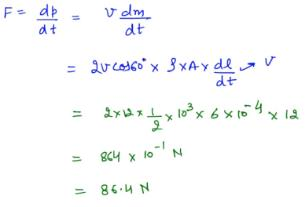QUESTION: 30

The cross sectional area of a horizontal tube increases along its length linearly, as we move in the direction of flow. The variation of pressure, as we move along its length in the direction of flow (x-direction), is best depicted by which of the following graphs

Solution: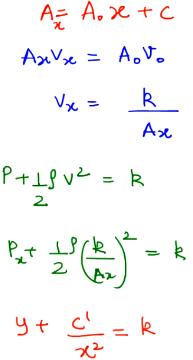So, it will be a downward facing parabola.